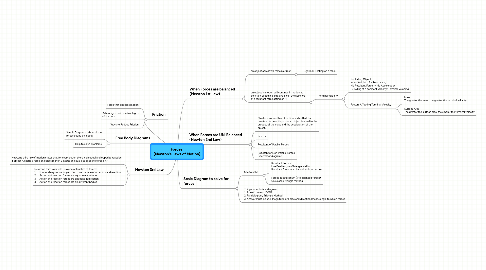# Forces (Newton's Laws of Motion)

Get Started. It's Free
or sign up with your email addressForces (Newton's Laws of Motion)## 1. When Forces are balanced (Newton 1st Law)

### 1.1. an object at rest will remain at rest

1.1.1. E.g: Book Resting on a table

### 1.2. an object in motion will continue in motion at constant speed in a straight line in the absence of a resultant force acting on it.

1.2.1. Terminal Velocity

1.2.1.1. For Falling Object, when Weight = Air Resistance, No Resultant Force -> No Acceleration -> Falling at a constant velocity (Terminal Velocity)

1.2.1.2. Factors Affecting Terminal Velocity

1.2.1.2.1. Mass The greater the mass, the greater the terminal velocity

1.2.1.2.2. Surface Area The greater the surface area, the smaller the terminal velocity

## 6. Scale Diagram to solve for forces

### 6.1. Use to solve

6.1.1. Resultant Forces Use Parallelogram/Triangle Method Resultant force must have double arrow head

6.1.2. Forces in Equilibrium ( No balanced forces) Use closed triangle method